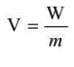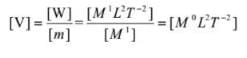NEET  >  Test: Gravitational Potential Energy

# Test: Gravitational Potential Energy

Test Description

## 20 Questions MCQ Test Physics Class 11 | Test: Gravitational Potential Energy

Test: Gravitational Potential Energy for NEET 2022 is part of Physics Class 11 preparation. The Test: Gravitational Potential Energy questions and answers have been prepared according to the NEET exam syllabus.The Test: Gravitational Potential Energy MCQs are made for NEET 2022 Exam. Find important definitions, questions, notes, meanings, examples, exercises, MCQs and online tests for Test: Gravitational Potential Energy below.
Solutions of Test: Gravitational Potential Energy questions in English are available as part of our Physics Class 11 for NEET & Test: Gravitational Potential Energy solutions in Hindi for Physics Class 11 course. Download more important topics, notes, lectures and mock test series for NEET Exam by signing up for free. Attempt Test: Gravitational Potential Energy | 20 questions in 20 minutes | Mock test for NEET preparation | Free important questions MCQ to study Physics Class 11 for NEET Exam | Download free PDF with solutions
 1 Crore+ students have signed up on EduRev. Have you?
Test: Gravitational Potential Energy - Question 1

### Weight is

Detailed Solution for Test: Gravitational Potential Energy - Question 1

Weight is a force caused by gravity. The weight of an object is the gravitational force between the object and the Earth. The more mass the object has the greater its weight will be. Weight is a force, so it's measured in newtons.

Test: Gravitational Potential Energy - Question 2

### When a satellite moves in a circular orbit, the _______acceleration is provided by the gravitational attraction of the earth

Detailed Solution for Test: Gravitational Potential Energy - Question 2

When any body or particle moves in circular orbit centripetal force acts on it and to move the object centripetal acceleration is necessary. So, when a satellite moves in circular orbit the centripetal acceleration is provided by the gravitational attraction of the earth.

Test: Gravitational Potential Energy - Question 3

### An unmanned space probe is to the thrown with such a velocity that it does not return to earth. It should be thrown with

Detailed Solution for Test: Gravitational Potential Energy - Question 3

It should be thrown with a velocity greater than or equal to escape velocity of the planet only then it will not return to Earth
Escape Velocity = √(2GM/R) = (2GM/R)1/2
Hence A is the correct answer.

Test: Gravitational Potential Energy - Question 4

What could be the maximum value for gravitational potential energy?

Detailed Solution for Test: Gravitational Potential Energy - Question 4

As, U=−Gm1m2/r,
it can be used to describe the potential energy in a system of point charges (or radially symmetric spheres) as a function of their separation distance r, then the maximum value is zero at infinite separation.
Hence, its maximum value is zero at infinity.

Test: Gravitational Potential Energy - Question 5

The value of escape velocity on earth when the body is thrown making an angle of 45o with the horizontal is

Detailed Solution for Test: Gravitational Potential Energy - Question 5

Escape velocity does not depend on the angle of projection. Escape velocity will remain the same.
Hence, escape velocity is 11.2km/s .

Test: Gravitational Potential Energy - Question 6

The escape velocity for the moon is nearly

Detailed Solution for Test: Gravitational Potential Energy - Question 6

In common usage, the initial point is on the surface of a planet or moon. On the surface of the Earth, the escape velocity is about 11.2 km/s, which is approximately 33 times the speed of sound (Mach 33) and several times the muzzle velocity of a rifle bullet (up to 1.7 km/s).

Test: Gravitational Potential Energy - Question 7

A body is projected with velocity ‘v’ from a planet with escape velocity ‘ve‘ .What will happen if v < ve

Detailed Solution for Test: Gravitational Potential Energy - Question 7

If velocity of satellite is is less than tge escape velocity then the satellite can go to certain hight and then cames back because the satellite is under the gravitational influence. therefore gravitational force acting on the satellite and due to this force satellite camse back

Test: Gravitational Potential Energy - Question 8

If r represents the radius of the orbit of the satellite of mass m moving around planet of mass M the velocity v of satellite is given by

Test: Gravitational Potential Energy - Question 9

Escape velocity is:

Detailed Solution for Test: Gravitational Potential Energy - Question 9

Escape velocity is the minimum velocity with which the body has to be projected vertically upwards from the surface of the earth so that it crosses the gravitational field of earth and never returns back on its own.

Test: Gravitational Potential Energy - Question 10

The gravitational potential due to the gravitational force on the earth is defined as the

Detailed Solution for Test: Gravitational Potential Energy - Question 10

Gravitational Potential is dened as the potential energy of a particle of unit mass at that point due to the gravitational force exerted byearth. Gravitational potential energy of a unit mass is known as gravitational potential.

Test: Gravitational Potential Energy - Question 11

A boy can jump 2 m on the surface of earth. How much can he jump on the surface of moon assuming that g on moon is 1/5 that of earth.

Detailed Solution for Test: Gravitational Potential Energy - Question 11

so, we see at the top most point the velocity the boy is zero and the earth's pull is acting downwards.
Given, gm/ge=1/6
=>ge=6gm
So using,
v² = u² - 2gs (where g=acceleration due to gravity, s=total height)
so,
0 = u² - 2gs
⇒ s=u2/2ge
⇒ 2=u2/2ge
Putting ge =6gm
⇒ 2=u2/gm
⇒ 12=u2/2gm
so the boy can jump a height of 12 m on the moon's surface.

Test: Gravitational Potential Energy - Question 12

The mass of the moon is 1/81 of earth’s mass and its radius 1/4 that of earth. If the escape velocity from the earth’s surface is 11.2 km/s ,its value for the moon…

Test: Gravitational Potential Energy - Question 13

Velocity of geostationary satellite with respect to earth is

Detailed Solution for Test: Gravitational Potential Energy - Question 13

Because geostationary satellite ( completes it's rotation in about 24hrs).and it's rotation cycle is same as that of Earth .thus it's velocity is zero with respect to earth

Test: Gravitational Potential Energy - Question 14

What is gravitational potential?

Detailed Solution for Test: Gravitational Potential Energy - Question 14

The gravitational potential at a point is defined as the work done in bringing the unit mass from infinity to that point without acceleration.Gravitational potential is a scalar quantity. It is denoted by V. Its S.I. unit is J kg^-1. Its CGS unit is erg g-1Dimensions of gravitational potential are [M0L2T-2].

Test: Gravitational Potential Energy - Question 15

Gravitational Potential is:

Detailed Solution for Test: Gravitational Potential Energy - Question 15

Gravitational Potential.Gravitational potential is the potential energy per kilogram at a point in a field. So the units are Jkg-1, joules per kilogram.The equation for the potential: = -Gm/r.Potential is not a vector even though it has a -ve sign. It doesn't have direction only magnitude. So, it is scalar quantity.Hence, correct answer is (A).

Test: Gravitational Potential Energy - Question 16

An earth satellite is moved from one stable circular orbit to a farther stable circular orbit. which one of following quantity increases

Detailed Solution for Test: Gravitational Potential Energy - Question 16

We know that gravitational potential is negative in sign and its magnitude decreases when distance from the massive attracting object increases, hence when considered with sign we can say that gravitational potential increases with increases in distance.

Test: Gravitational Potential Energy - Question 17

What does the term escape speed of a planet mean?

Detailed Solution for Test: Gravitational Potential Energy - Question 17

Escape velocity is the minimum velocity with which the body has to be projected vertically upwards from the surface of the earth so that it crosses the gravitational field of earth and never returns back on its own.

Test: Gravitational Potential Energy - Question 18

Earth attracts a body with a force equal to its

Detailed Solution for Test: Gravitational Potential Energy - Question 18

Weight is a consequence of the universal law of gravitation: any two objects, because of their masses, attract each other with a force that is directly proportional to the product of their masses and inversely proportional to the square of the distance between them. A mass is pulled towards the earth with “g” and thus comprises the weight of that body.

Test: Gravitational Potential Energy - Question 19

Calculate the escape velocity on the surface of the planet of 5.3 x 1020 grams, with radius 1.3 x 105 m.

Test: Gravitational Potential Energy - Question 20

The energy required to be spent by a satellite of mass m and speed v and orbital radius r in completing a circular orbit once round the earth of mass M is

Detailed Solution for Test: Gravitational Potential Energy - Question 20

As the satellite is bounded by earth's gravitational field and has some velocity, it would completely revolve around the circular orbit without any external energy required.

## Physics Class 11

126 videos|449 docs|210 tests
 Use Code STAYHOME200 and get INR 200 additional OFF Use Coupon Code
Information about Test: Gravitational Potential Energy Page
In this test you can find the Exam questions for Test: Gravitational Potential Energy solved & explained in the simplest way possible. Besides giving Questions and answers for Test: Gravitational Potential Energy, EduRev gives you an ample number of Online tests for practice

## Physics Class 11

126 videos|449 docs|210 tests

### How to Prepare for NEET

Read our guide to prepare for NEET which is created by Toppers & the best Teachers